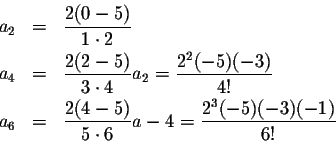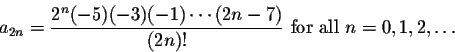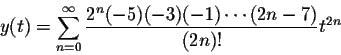## Series Solutions: Hermite's Equation#### Exercise 3:

Consider the Hermite Equation of order 5:

y''-2ty'+10y=0.

Find the solution satisfying the initial conditions a0=1, a1=0.

The solution will not be a polynomial.

Since a1=0, all odd coefficients will be zero. Let's compute a few of the even coefficients:From this it is not too hard to come up with the general formula:This leads to the formulafor the solution to the given initial value problem.

The solution converges and solves the equation for all real numbers.[Back] [Exercises] [Next]
[Algebra] [Trigonometry] [Complex Variables]
[Calculus] [Differential Equations] [Matrix Algebra]S.O.S MATHematics home page

Do you need more help? Please post your question on our S.O.S. Mathematics CyberBoard.Helmut Knaust
1998-07-08